# medfreq

Median frequency

## Syntax

``freq = medfreq(x)``
``freq = medfreq(x,fs)``
``freq = medfreq(pxx,f)``
``freq = medfreq(sxx,f,rbw)``
``freq = medfreq(___,freqrange)``
``````[freq,power] = medfreq(___)``````
``medfreq(___)``

## Description

````freq = medfreq(x)` estimates the median normalized frequency, `freq`, of the power spectrum of a time-domain signal, `x`.```

example

````freq = medfreq(x,fs)` estimates the median frequency in terms of the sample rate, `fs`.```

example

````freq = medfreq(pxx,f)` returns the median frequency of a power spectral density (PSD) estimate, `pxx`. The frequencies, `f`, correspond to the estimates in `pxx`.```
````freq = medfreq(sxx,f,rbw)` returns the median frequency of a power spectrum estimate, `sxx`, with resolution bandwidth `rbw`.```
````freq = medfreq(___,freqrange)` specifies the frequency interval over which to compute the median frequency. This syntax can include any combination of input arguments from previous syntaxes, as long as the second input argument is either `fs` or `f`. If the second input is passed as empty, normalized frequency will be assumed. The default value for `freqrange` is the entire bandwidth of the input signal.```

example

``````[freq,power] = medfreq(___)``` also returns the band power, `power`, of the spectrum. If you specify `freqrange`, then `power` contains the band power within `freqrange`.```
````medfreq(___)` with no output arguments plots the PSD or power spectrum and annotates the median frequency.```

## Examples

collapse all

Generate 1024 samples of a chirp sampled at 1024 kHz. Specify the chirp so that it has an initial frequency of 50 kHz and reaches 100 kHz at the end of the sampling. Add white Gaussian noise such that the signal-to-noise ratio is 40 dB. Reset the random number generator for reproducible results.

```nSamp = 1024; Fs = 1024e3; SNR = 40; rng default t = (0:nSamp-1)'/Fs; x = chirp(t,50e3,nSamp/Fs,100e3); x = x+randn(size(x))*std(x)/db2mag(SNR);```

Estimate the median frequency of the chirp. Plot the power spectral density (PSD) and annotate the median frequency.

`medfreq(x,Fs)`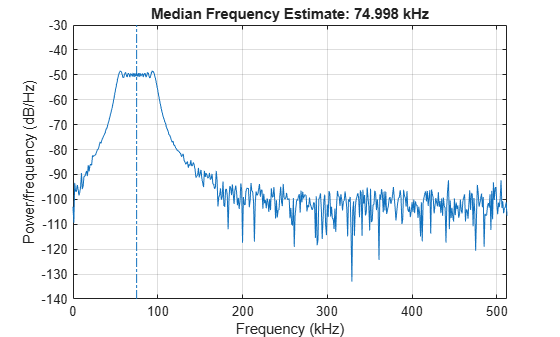```ans = 7.4998e+04 ```

Generate another chirp. Specify an initial frequency of 200 kHz, a final frequency of 300 kHz, and an amplitude that is twice that of the first signal. Add white Gaussian noise.

```x2 = 2*chirp(t,200e3,nSamp/Fs,300e3); x2 = x2+randn(size(x2))*std(x2)/db2mag(SNR);```

Concatenate the chirps to produce a two-channel signal. Estimate the median frequency of each channel.

`y = medfreq([x x2],Fs)`
```y = 1×2 105 × 0.7500 2.4999 ```

Plot the PSDs of the two channels and annotate their median frequencies.

`medfreq([x x2],Fs);`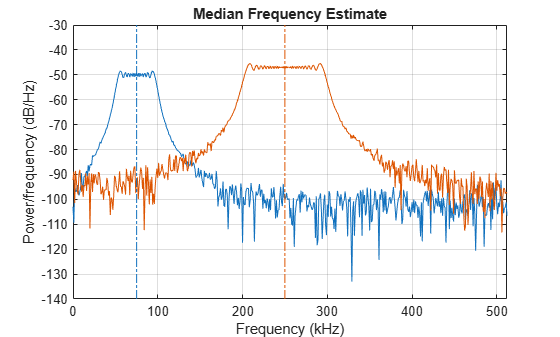Add the two channels to form a new signal. Plot the PSD and annotate the median frequency.

`medfreq(x+x2,Fs)`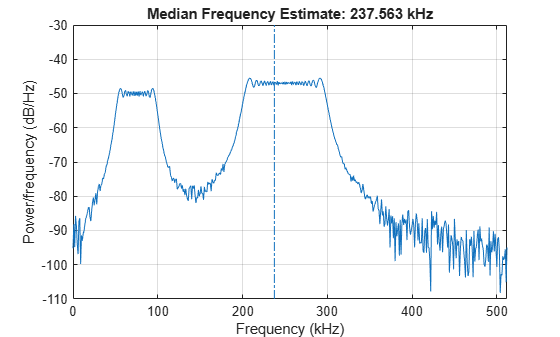```ans = 2.3756e+05 ```

Generate 1024 samples of a 100.123 kHz sinusoid sampled at 1024 kHz. Add white Gaussian noise such that the signal-to-noise ratio is 40 dB. Reset the random number generator for reproducible results.

```nSamp = 1024; Fs = 1024e3; SNR = 40; rng default t = (0:nSamp-1)'/Fs; x = sin(2*pi*t*100.123e3); x = x + randn(size(x))*std(x)/db2mag(SNR);```

Use the `periodogram` function to compute the power spectral density (PSD) of the signal. Specify a Kaiser window with the same length as the signal and a shape factor of 38. Estimate the median frequency of the signal and annotate it on a plot of the PSD.

```[Pxx,f] = periodogram(x,kaiser(nSamp,38),[],Fs); medfreq(Pxx,f);```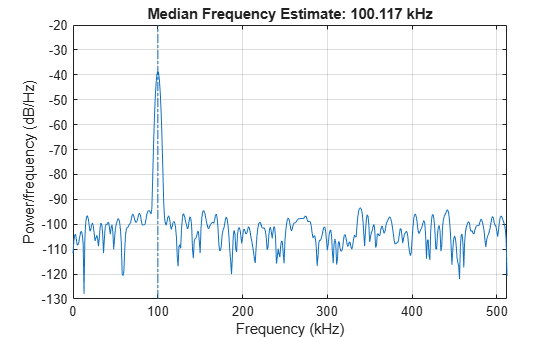Generate another sinusoid, this one with a frequency of 257.321 kHz and an amplitude that is twice that of the first sinusoid. Add white noise.

```x2 = 2*sin(2*pi*t*257.321e3); x2 = x2 + randn(size(x2))*std(x2)/db2mag(SNR);```

Concatenate the sinusoids to produce a two-channel signal. Estimate the PSD of each channel and use the result to determine the median frequency.

```[Pyy,f] = periodogram([x x2],kaiser(nSamp,38),[],Fs); y = medfreq(Pyy,f)```
```y = 1×2 105 × 1.0012 2.5731 ```

Annotate the median frequencies of the two channels on a plot of the PSDs.

`medfreq(Pyy,f);`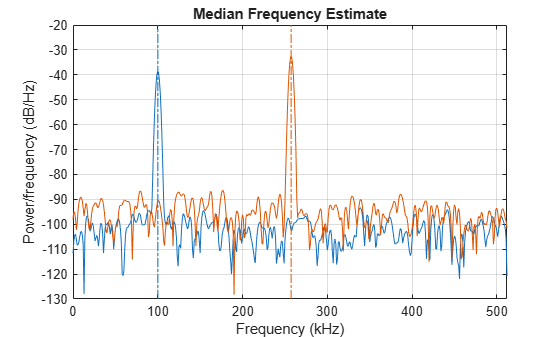Add the two channels to form a new signal. Estimate the PSD and annotate the median frequency.

```[Pzz,f] = periodogram(x+x2,kaiser(nSamp,38),[],Fs); medfreq(Pzz,f);```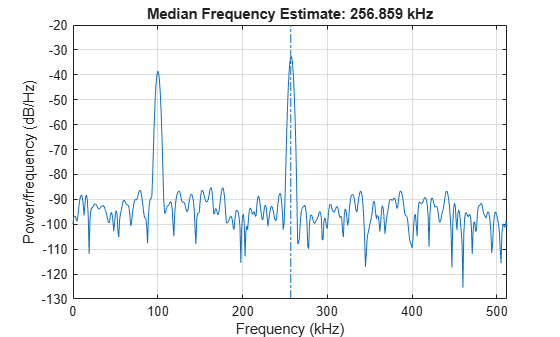Generate a signal whose PSD resembles the frequency response of an 88th-order bandpass FIR filter with normalized cutoff frequencies $0.25\pi$ rad/sample and $0.45\pi$ rad/sample.

`d = fir1(88,[0.25 0.45]);`

Compute the median frequency of the signal between $0.3\pi$ rad/sample and $0.6\pi$ rad/sample. Plot the PSD and annotate the median frequency and measurement interval.

`medfreq(d,[],[0.3 0.6]*pi);`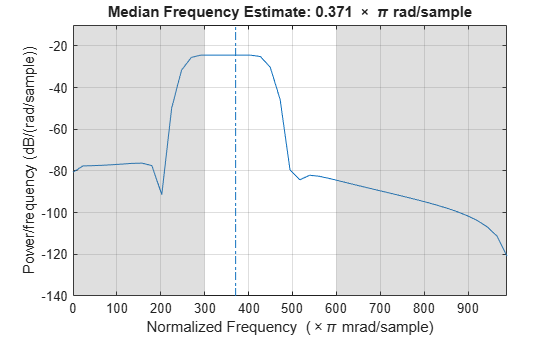Output the median frequency and the band power of the measurement interval. Specifying a sample rate of $2\pi$ is equivalent to leaving the rate unset.

```[mdf,power] = medfreq(d,2*pi,[0.3 0.6]*pi); fprintf('Mean = %.3f*pi, power = %.1f%% of total \n', ... mdf/pi,power/bandpower(d)*100)```
```Mean = 0.371*pi, power = 77.4% of total ```

Add a second channel with normalized cutoff frequencies $0.5\pi$ rad/sample and $0.8\pi$ rad/sample and an amplitude that is one-tenth that of the first channel.

`d = [d;fir1(88,[0.5 0.8])/10]';`

Compute the median frequency of the signal between $0.3\pi$ rad/sample and $0.9\pi$ rad/sample. Plot the PSD and annotate the median frequency of each channel and the measurement interval.

`medfreq(d,[],[0.3 0.9]*pi);`Output the median frequency of each channel. Divide by $\pi$.

`mdf = medfreq(d,[],[0.3 0.9]*pi)/pi`
```mdf = 1×2 0.3706 0.6500 ```

## Input Arguments

collapse all

Input signal, specified as a vector or matrix. If `x` is a vector, it is treated as a single channel. If `x` is a matrix, then `medfreq` computes the median frequency of each column of `x` independently. `x` must be finite-valued.

Example: `cos(pi/4*(0:159))+randn(1,160)` is a single-channel row-vector signal.

Example: `cos(pi./[4;2]*(0:159))'+randn(160,2)` is a two-channel signal.

Data Types: `single` | `double`

Sample rate, specified as a positive real scalar. The sample rate is the number of samples per unit time. If the time is measured in seconds, then the sample rate is in hertz.

Data Types: `single` | `double`

Power spectral density (PSD), specified as a vector or matrix. If `pxx` is a matrix, then `medfreq` computes the median frequency of each column of `pxx` independently.

The power spectral density must be expressed in linear units, not decibels. Use `db2pow` to convert decibel values to power values.

Example: ```[pxx,f] = periodogram(cos(pi./[4;2]*(0:159))'+randn(160,2))``` specifies the periodogram PSD estimate of a noisy two-channel sinusoid sampled at 2π Hz and the frequencies at which it is computed.

Data Types: `single` | `double`

Frequencies, specified as a vector.

Data Types: `single` | `double`

Power spectrum estimate, specified as a vector or matrix. If `sxx` is a matrix, then `medfreq` computes the median frequency of each column of `sxx` independently.

The power spectrum must be expressed in linear units, not decibels. Use `db2pow` to convert decibel values to power values.

Example: ```[sxx,w] = periodogram(cos(pi./[4;2]*(0:159))'+randn(160,2),'power')``` specifies the periodogram power spectrum estimate of a two-channel sinusoid embedded in white Gaussian noise and the normalized frequencies at which it is computed.

Data Types: `single` | `double`

Resolution bandwidth, specified as a positive scalar. The resolution bandwidth is the product of two values: the frequency resolution of the discrete Fourier transform and the equivalent noise bandwidth of the window used to compute the PSD.

Data Types: `single` | `double`

Frequency range, specified as a two-element vector of real values. If you do not specify `freqrange`, then `medfreq` uses the entire bandwidth of the input signal.

Data Types: `single` | `double`

## Output Arguments

collapse all

Median frequency, specified as a scalar or vector.

• If you specify a sample rate, then `freq` has the same units as `fs`.

• If you do not specify a sample rate, then `freq` has units of rad/sample.

Band power, returned as a scalar or vector.